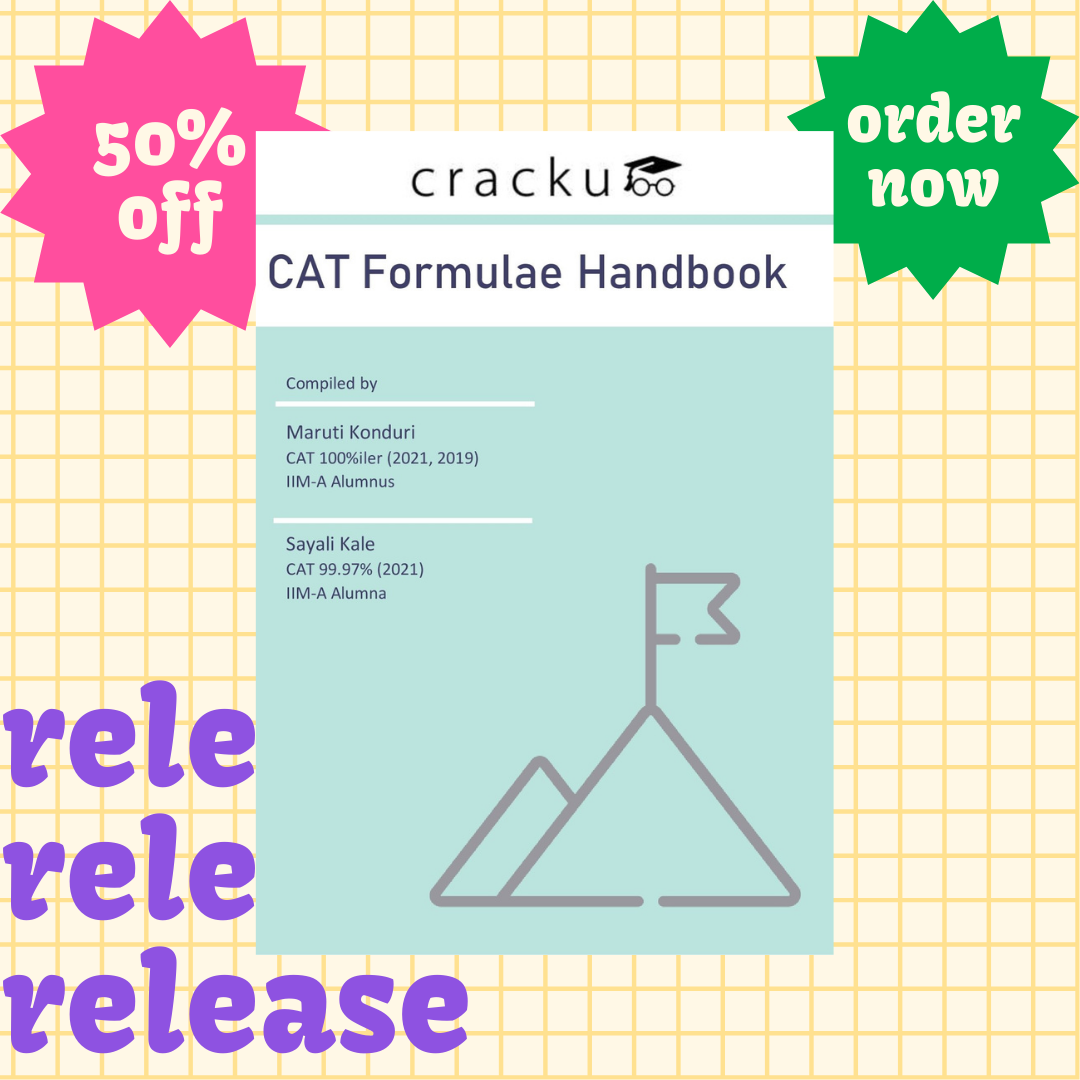# Set theory and Venn diagrams formulas for CAT PDF

0
50547

Sets and Venn diagrams is one of the easiest topics of CAT. Most of the Set Thoery formulas of CAT in this section can be deduced logically with little effort. The emphasis should be made while translating the sentences into areas of the Venn diagram. While solving, pay careful attention to phrases like and, or, not, only, in as these generally signify the relationship.

Looking for hardcopy handbook?

Order below. Delivery charges are on us 🙂Outline of things covered in this sets and venn diagrams formulas  for CAT pdf are:

• Definition of a set
• Elements of the set-Cardinal Number
• Types of Sets (Null set, Singleton set, Finite and Infinite set, Universal set, Subset, Equal sets, Disjoint sets, Power set)
• Properties of Sets
• Definition and concepts on Venn diagrams
• Union and Intersection of two or more sets
• Formulas for Venn diagrams
• Maximizing and Minimizing Overlap
• Some other important properties for Sets and Venn diagrams

Download All Quantitative aptitude formulas of CAT. Just learning Set theory formulas for CAT is not enough, practice is more important for exams like CAT, so try this practice questions for CAT.

Taking a free CAT mock test to analyse your score and get a national level percentile.